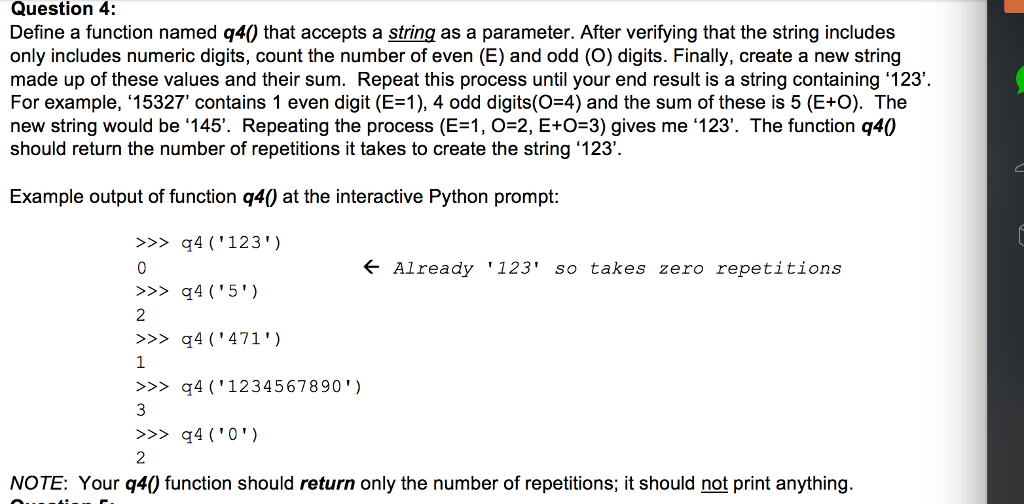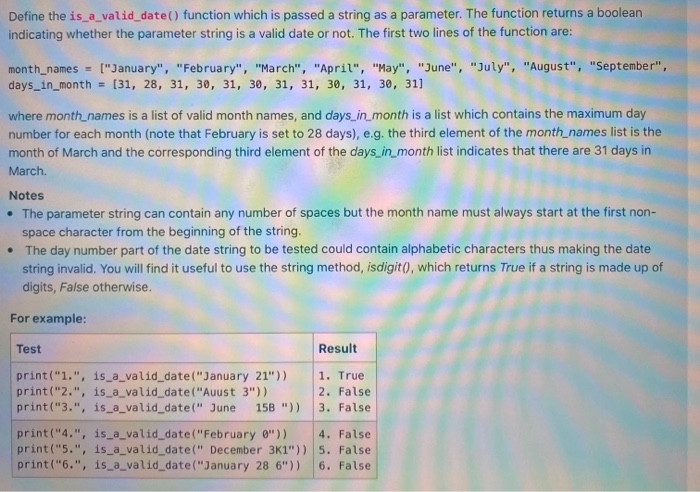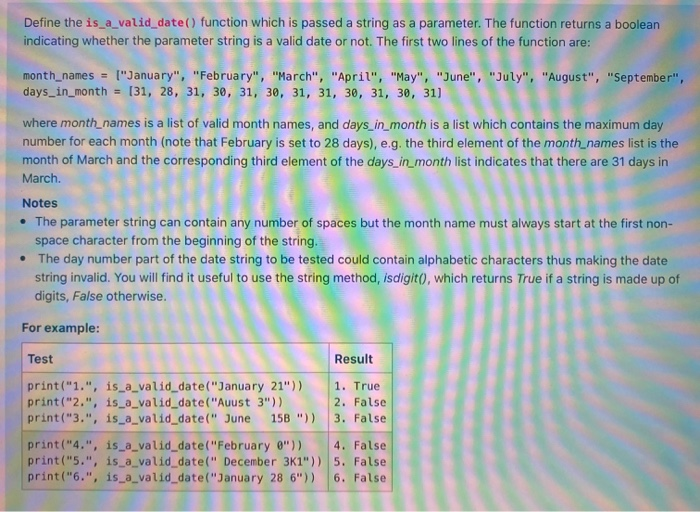# In the function below, return the string 'negative' if the numeric parameter number is in fact...

In the function below, return the string 'negative' if the numeric parameter number is in fact negative, or, return the string 'positive' if the parameter number is positive, otherwise return string 'zero'

use function "def string_indicating_positivityOrNegativity(number):"

python 3

```def string_indicating_positivityOrNegativity(number):
if number<0:
return 'negative'
elif number>0:
return 'positive'
else:
return 'zero'```

#### Earn Coin

Coins can be redeemed for fabulous gifts.

Similar Homework Help Questions
• ### HW7.26. Return a CSV string from a list of numbers The function below takes a single...

HW7.26. Return a CSV string from a list of numbers The function below takes a single parameter, a list of numbers called number_list. Complete the function to return a string of the provided numbers as a series of comma separate values (CSV). For example, if the function was provided the argument [22, 33, 44], the function should return '22,33,44'. Hint: in order to use the join function you need to first convert the numbers into strings, which you can do...

• ### python function will Return True if string x contains 3 vowels in a row, in consecutive...

python function will Return True if string x contains 3 vowels in a row, in consecutive locations, false otherwise. assuming that 'vowels' refer to the following lowercase lttrs: a,e,i,o,u programs fails partially, only allowed to use float, str, int, appen, split, strip, len, range def vowels_three(x): for i in range (o, len(x), 2): if x[i] not in ('a,e,i,o,u'): return False return True

• ### Write a Python program which defines a function named "divide_two_numbers" that accepts two numeric parameters and...

Write a Python program which defines a function named "divide_two_numbers" that accepts two numeric parameters and divides the first parameter by the second. Your divide_two_numbers function must check the second parameter before dividing; if it is zero, raise an ZeroDivisionError exception which includes the string "second parameter was zero!" as a parameter instead of processing the division. Your main function must call your divide_two_numbers function at least twice; one call must use two normal parameters to verify normal operation, the...

• ### please use python Question 4: Define a function named q40 that accepts a string as a...please use python Question 4: Define a function named q40 that accepts a string as a parameter. After verifying that the string includes only includes numeric digits, count the number of even (E) and odd (O) digits. Finally, create a new string made up of these values and their sum. Repeat this process until your end result is a string containing 123 For example, '15327' contains 1 even digit (E-1), 4 odd digits(O-4) and the sum of these is 5...

• ### (7) Implement the shorten_space() function. shorten_space() has a string parameter and updates the string by replacing...

(7) Implement the shorten_space() function. shorten_space() has a string parameter and updates the string by replacing all sequences of 2 or more spaces with a single space. shorten_space() returns the string. Call shorten_space() in the print_menu() function, and then output the edited string. Hint: Look up and use Python function .isspace(). Ex: Edited text: we'll continue our quest in space. there will be more shuttle flights and more shuttle crews and, yes, more volunteers, more civilians, more teachers in space....

• ### Define the is_a_valid_date() function which is passed a string as a parameter. The function returns a...Define the is_a_valid_date() function which is passed a string as a parameter. The function returns a boolean indicating whether the parameter string is a valid date or not. The first two lines of the function are: month_names = ["January", "February", "March", "April", "May", "June", "July", "August", "September", days_in_month = [31, 28, 31, 30, 31, 30, 31, 31, 30, 31, 30, 31] where month_names is a list of valid month names, and days_in_month is a list which contains the maximum day...

• ### Define the is_a_valid_date() function which is passed a string as a parameter. The function returns a...Define the is_a_valid_date() function which is passed a string as a parameter. The function returns a boolean indicating whether the parameter string is a valid date or not. The first two lines of the function are: month_names = ["January", "February", "March","April", "May", "June", "July", "August", "September", days_in_month = (31, 28, 31, 30, 31, 30, 31, 31, 30, 31, 30, 31) where month_names is a list of valid month names, and days_in_month is a list which contains the maximum day number...

• ### 7.10 Strings Implement the get_num_of_non_WS_characters() function. get_num_of_non_WS_characters() has a string parameter and returns the number of...

7.10 Strings Implement the get_num_of_non_WS_characters() function. get_num_of_non_WS_characters() has a string parameter and returns the number of characters in the string, excluding all whitespace. Implement the get_num_of_words() function. get_num_of_words() has a string parameter and returns the number of words in the string.

• ### 4. Write a C function int str2int(char *s) that takes string s as parameter and return...4. Write a C function int str2int(char *s) that takes string s as parameter and return the integer that s represents. For example, if string s is 123", then the function return integer 123.

• ### Python help! Any help is appreciated, thank you! Fill in the missing function, monthString(), in the...

Python help! Any help is appreciated, thank you! Fill in the missing function, monthString(), in the program. The function should take number between 1 and 12 as a parameter and returns the corresponding month as a string. For example, if the parameter is 1, your function should return "January". If the parameter is 2, your function should return out "February", etc. def monthString(monthNum): """ Takes as input a number, monthNum, and returns the corresponding month name as a string. Example:...